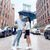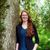Announcements
The way to achieve your own success is the willingness to help somebody else. Go for it!
cancel
Showing results for
Did you mean:Creator III

## Calculating Max/Min in a Moving Array

Hello everyone,

I'm trying to find a max value within a constantly moving array. The idea is very simple, I have a database with two fields - Date and Exchange Rate - and, for each row of data, I'm trying to find a highest and lowest exchange rate over the last year.

For example, I have an exchange rate for each day over the period of two years starting on Jan 1, 2015 and ending Dec 31, 2016. I would like to create a field in the script that would:

• Look at every date on each row of data
• Find a date one year from date on current row
• Search through an array of dates that is between date that is on specific row and date that is a year ago from date on a row
• Find max exchange rate for an array identified in point 3

Continuing with the example, on Jan 1, 2015, max exchange rate would be the exchange rate for Jan 1, 2015. For 2nd line of data that has an exchange rate for Jan 2, 2015, QlikView would look at an array of two dates and corresponding exchange rates and select the max exchange rate between Jan 1, 2015 and Jan 2, 2015. For the line of data that has, say Mar 14, 2016 date, it would look for an array of data from Mar 15, 2015 through Mar 14, 2016 and pick the max exchange rate (which should be 0.952744) for that one year period.

Attached is spreadsheet with exchange rates and QVW that I'm working on. In the QVW I'm trying to find a way to populate the 12_Months_High and 12_Months_Low fields.

Any help will be much much appreciated! Thanks in advance Qlik Community!

Mikhail Bespartochnyy

1 Solution

Accepted SolutionsMVP

This should do the trick:

```Table:
[USD/EUR] as Exchange_Rate
FROM
Exchange_Rates.xlsx
(ooxml, embedded labels, table is Exchange_Rates);

Table2:
If(RowNo() = 1, Null(), Previous(Exchange_Date)) as Previous_Date,
Exchange_Rate,
If(RowNo() = 1, Null(), Previous(Exchange_Rate)) as Previous_Exchange_Rate
Resident Table
Order By Exchange_Date;

DROP Table Table;

Table3:
Previous_Date,
Exchange_Rate,
Previous_Exchange_Rate,
Num((Exchange_Rate / Previous_Exchange_Rate) - 1, '###.##%') as Percent_Change//,
//'' as [12_Months_High],
//'' as [12_Months_Low]
Resident Table2;

DROP Table Table2;

FOR i=0 TO NoOfRows('Table3')
Temp:
LOAD PEEK('Exchange_Date', \$(i), 'Table3') AS Exchange_Date,
MAX(Exchange_Rate) AS [12_Months_High],
MIN(Exchange_Rate) AS [12_Months_Low]
RESIDENT Table3
WHERE Exchange_Date > AddYears(PEEK('Exchange_Date', \$(i), 'Table3'), -1)
AND Exchange_Date <= PEEK('Exchange_Date', \$(i), 'Table3');
NEXT

LEFT JOIN (Table3)
RESIDENT Temp;

DROP TABLE Temp;
```

I've also attached a working copy of your example file.

10 RepliesMVP

This should do the trick:

```Table:
[USD/EUR] as Exchange_Rate
FROM
Exchange_Rates.xlsx
(ooxml, embedded labels, table is Exchange_Rates);

Table2:
If(RowNo() = 1, Null(), Previous(Exchange_Date)) as Previous_Date,
Exchange_Rate,
If(RowNo() = 1, Null(), Previous(Exchange_Rate)) as Previous_Exchange_Rate
Resident Table
Order By Exchange_Date;

DROP Table Table;

Table3:
Previous_Date,
Exchange_Rate,
Previous_Exchange_Rate,
Num((Exchange_Rate / Previous_Exchange_Rate) - 1, '###.##%') as Percent_Change//,
//'' as [12_Months_High],
//'' as [12_Months_Low]
Resident Table2;

DROP Table Table2;

FOR i=0 TO NoOfRows('Table3')
Temp:
LOAD PEEK('Exchange_Date', \$(i), 'Table3') AS Exchange_Date,
MAX(Exchange_Rate) AS [12_Months_High],
MIN(Exchange_Rate) AS [12_Months_Low]
RESIDENT Table3
WHERE Exchange_Date > AddYears(PEEK('Exchange_Date', \$(i), 'Table3'), -1)
AND Exchange_Date <= PEEK('Exchange_Date', \$(i), 'Table3');
NEXT

LEFT JOIN (Table3)
RESIDENT Temp;

DROP TABLE Temp;
```

I've also attached a working copy of your example file.MVP

Another option

Table:

[USD/EUR] as Exchange_Rate

FROM

Exchange_Rates.xlsx

(ooxml, embedded labels, table is Exchange_Rates);

Table2:

If(RowNo() = 1, Null(), Previous(Exchange_Date)) as Previous_Date,

Exchange_Rate,

If(RowNo() = 1, Null(), Previous(Exchange_Rate)) as Previous_Exchange_Rate,

Num((Exchange_Rate / If(RowNo() = 1, Null(), Previous(Exchange_Rate))) - 1, '###.##%') as Percent_Change

Resident Table

Order By Exchange_Date;

DROP Table Table;

Left Join (Table2)

LOAD Date(Exchange_Date + IterNo() - 1) as Exchange_Date,

Exchange_Date as AsOfExchangeDate,

Exchange_Rate as NewExchange_Rate

Resident Table2

While IterNo() <= 367;

FinalTable:

Previous_Date,

Exchange_Rate,

Previous_Exchange_Rate,

Percent_Change,

Max(NewExchange_Rate) as [12_Month_High],

Min(NewExchange_Rate) as [12_Month_Low]

Resident Table2

Group By Exchange_Date, Previous_Date, Exchange_Rate, Previous_Exchange_Rate, Percent_Change;

DROP Table Table2;Creator III
Author

Thanks, Nicole! This works, but I'm struggling to fully understand how. In your FOR NEXT loop is QlikView creating a line of data in Temp table and then at each loop it's concatenating a new line to Temp table and it's doing that without Concatenate function?MVP

When field names are the same, the table get Auto-Concatenated unless you have NoConcatenate to force them not to concatenateCreator III
Author

Ohhhhhh that's right! I've been breaking my head over that the whole afternoon. So technically each loop creates one table, but because there are no variation in field names, instead of creating 700-some tables the built-in function concatenates the data into one table to create 700-some rows in a single table instead. This get's me every time. I think I learned my lesson now. Thanks, Sunny!MVP

Yup, you are rightCreator III
Author

Thanks for the alternative option, Sunny! I added an if statement into While loop to variate number of days based on whether exchange date is in a leap year or not, but this one works just as well. Attached is the app with revised script.

P.S. Thanks for moving up Percent_Change to Table2Saved an extra resident load! It slipped my mind that it can be done there too.MVP

What you have is fairly complex, I would just use this:

While Exchange_Date + IterNo() - 1 <= AddYears(Exchange_Date, 1);Creator III
Author

Clever! I like that. Thanks again, Sunny!Community Browser